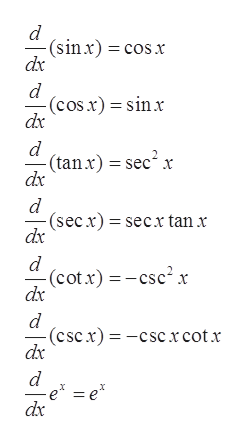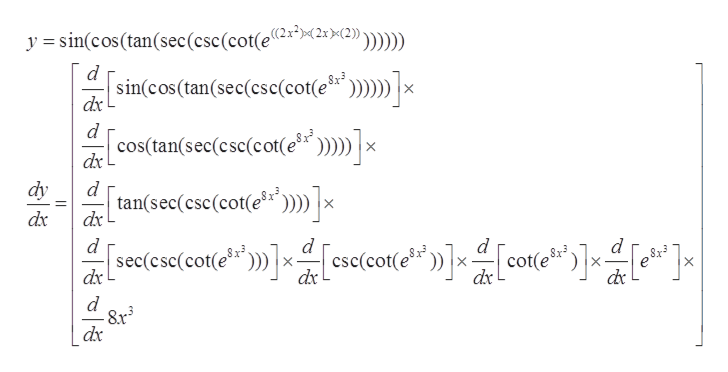# Using the chain rule find dy/dx for the function:y=sin(cos(tan(sec(csc(cot(e^((2x^2)(2x)(2)))))))))

Question

Using the chain rule find dy/dx for the function:

y=sin(cos(tan(sec(csc(cot(e^((2x^2)(2x)(2)))))))))

check_circleExpert Solution
Step 1

Given:

Step 2

Formula used:help_outlineImage Transcriptionclose(sinx) cosx dx d (cos.x) sinx dx d (tan x) sec2 x dx (secx) secx tan x dx d (cot x)csc2x dx -(cscx)-cscxcotx dx 11 d e =e* dx fullscreen
Step 3

So, using chain rule the derivative of ‘y&rsquo...help_outlineImage Transcriptionclose>(2)) y =sin(cos(tan(sec(csc (cot(e2x*>2xX2} Sx3 '»]x sincos(tan(sec(csc(cot(e dx d cos(tan(sec(csc(cot(e? x dx dy d tan(sec(csc(cot(e dx x dx d d cot(e dx \$x 8x3 dx dx d dx | fullscreen

### Want to see the full answer?

See Solution

#### Want to see this answer and more?

Solutions are written by subject experts who are available 24/7. Questions are typically answered within 1 hour*

See Solution
*Response times may vary by subject and question
Tagged in

### Other